## A binary search is to be performed on the list: 1 , 5 ,10 , 13 , 48 , 68 , 100 , 101 How many comparisons would it take to the find the numb

Question

A binary search is to be performed on the list: 1 , 5 ,10 , 13 , 48 , 68 , 100 , 101 How many comparisons would it take to the find the number 101?

in progress 0
5 months 2021-09-03T09:09:47+00:00 1 Answers 92 views 0

3 comparisons

Step-by-step explanation:

Given: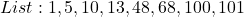Required

Determine the number of comparisons to get to 101

The length of the list is 8. So, the first index is 0 and the last is 7.

We have: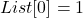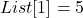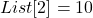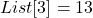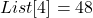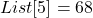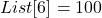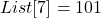Initially: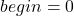— first index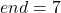— last index

Start by calculating the mid-item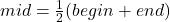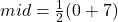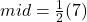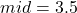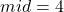— approximated.

The mid-index is 4 and the item is:101 is on the right of 48.

So, we calculate the new begin index.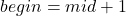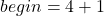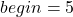Calculate mid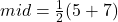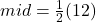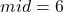The mid-index is 6 and the item is:101 is on the right of 100.

So, we calculate the new begin index.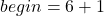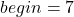Calculate mid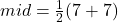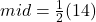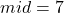At this point, the mid-index is at 101

i.e.This implies that, we have located 101.

So far, we made 3 comparisons